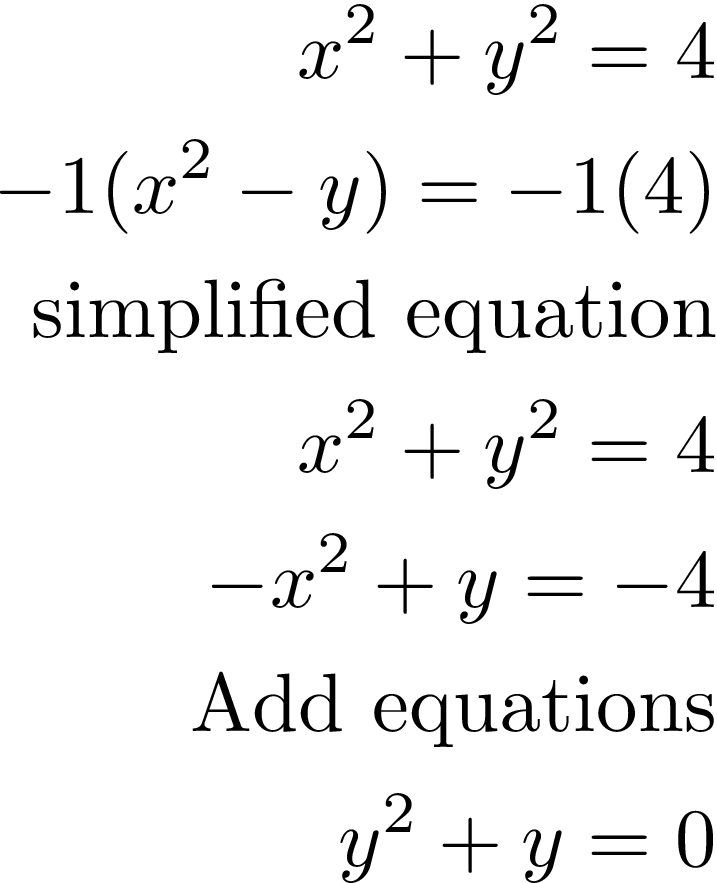# Solving a System of Non-Linear Equations

Solving a system of non-linear equations means that at least one of the equations is not linear. For example, if one equation has an exponent it may be a parabola or a circle. With this no shape that is not linear it involves slightly different expectations.

Solving a system of non-linear equations is similar to solving a system with linear equations with one difference. The difference is that with nonlinearequations you can have more than one solution. What this means is that the lines that are the equations can intersect in more than one place. However, it is also possible they do not intersect. How many solutions depends on the lines involved.

For example, if one equation is a circle/parabola and the other is a line there can be 0-2 solutions. If one equation makes a circle and the other makes a parabola there can be up to 4 solutions. Two circles or two parabolas can make a multitude of solutions

The steps for solving a nonlinear system are the same. Therefore, in this post, we will demonstrate how to solve a system of non-linear equations using the substitution and elimination methods.

Substitution

The substitution method is when we plug one equation into the variable of the other equation. Below is our system of equations.The first thing to notice is that the top equation would make a circle if you graphed it. That is why this is a non-linear system. To solve we take the second equation and substitute it for y. Below we find the values for xNow we complete the system by finding the values for y.THerefore are ordered pairs are (0, -3) and (1,0). These are the two points at which the equations intersect if you were to graph them.

Elimination

Elimination involves making the coefficients of one of the variables opposite so that when they are added together they cancel each other out. By removing one variable you can easily solve for the other. Below is the system of equation we want to solve.The top equation makes a circle while the bottom one makes a parabola. This means that we can have as many as four solutions for this system. To solve this system we will multiply the bottom equation by -1. This will allow us to remove the x variable and then solve for y. Below are the steps.Now we simply solve for y.Now we can take these values for y to solve for x.The order pairs are as follows

• (-2,0)
• (2,0)
•   (√3, -1)
• (-√3, -1)

Conclusion

From this, you can see that non-linear equations can be solved using the same approaches. Understanding this is key to many other fields of math such as data science and machine learning.